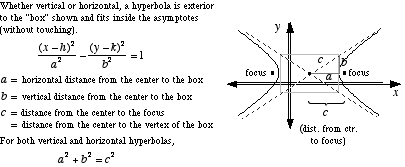# foci of a hyperbola

Foci of a Hyperbola

Two fixedpoints located inside each curve of a hyperbola that are used in the curve's formal definition. A hyperbola is defined as follows: For two given points, the foci, a hyperbola is the locus of points such that the difference between the distance to each focus is constant.